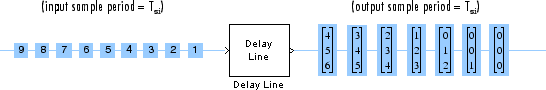Delay Line

Rebuffer sequence of inputs

Library

Signal Management / Buffers

dspbuff3

•Description

The Delay Line block rebuffers a sequence of Mi-by-N matrix inputs into a sequence of Mo-by-N matrix outputs, where Mo is the output frame size you specify in the Delay line size parameter. Depending on whether Mo is greater than, less than, or equal to the input frame size, Mi, the output frames can be underlapped or overlapped. The block always performs frame-based processing and rebuffers each of the N input channels independently.

When Mo > Mi, the output frame overlap is the difference between the output and input frame size, Mo-Mi. When Mo < Mi, the output is underlapped; the Delay Line block discards the first Mi-Mo samples of each input frame so that only the last Mo samples are buffered into the corresponding output frame. When Mo = Mi, the output data is identical to the input data, but is delayed by the latency of the block. Due to the block's latency, the outputs are always delayed by one frame, the entries of which you specify in the Initial conditions parameter (see Initial Conditions).

The output frame period is equal to the input frame period (Tfo=Tfi). The output sample period, Tso, is therefore equal to Tfi/Mo, or equivalently, Tsi(Mi/Mo)

In the most typical use, each output differs from the preceding output by only one sample, as illustrated below for scalar input.Note that the first output of the block in the example above is all zeros; this is because the Initial Conditions parameter is set to zero.

Initial Conditions

The Delay Line block's buffer is initialized to the value specified by the Initial conditions parameter. The block outputs this buffer at the first simulation step (t=0). When the block's output is a vector, the Initial conditions can be a vector of the same size, or a scalar value to be repeated across all elements of the initial output. When the block's output is a matrix, the Initial conditions can be a matrix of the same size, a vector (of length equal to the number of matrix rows) to be repeated across all columns of the initial output, or a scalar to be repeated across all elements of the initial output.

Examples

In the following ex_delayline_ref2 model, the block rebuffers a two-channel input with a Delay line size of 3.The first output frame in this example is due to the latency of the Delay Line block; it is all zeros because the Initial conditions parameter is set to zero. Because the input frame size of 4 is larger than the output frame size of 3, only the last three samples in each input frame are propagated to the corresponding output frame. The frame periods of the input and output are the same, and the output sample period is Tsi(Mi/Mo), or 4/3 the input sample period.

Parameters

Delay line size

Specify the number of rows in output matrix, Mo.

Initial conditions

Specify the value of the block's initial output. When the block outputs a vector, the Initial conditions can be a vector of the same size, or a scalar value to be repeated across all elements of the initial output. When the block outputs a matrix, the Initial conditions can be a matrix of the same size, a vector (of length equal to the number of matrix rows) to be repeated across all columns of the initial output, or a scalar to be repeated across all elements of the initial output.

Allow direct feedthrough

When you select this check box, the input data is not delayed by an extra frame before it is available at the output buffer. Instead, the input data is available immediately at the output port of the block.

Show En_Out port for selectively enabling output

When you select this check box, the En_Out port appears on the block icon. This block uses a circular buffer internally even though the output is linear. This means that for valid output, data from the circular buffer has to be linearized. The En_Out port determines whether or not a valid output needs to be computed based on the value of its Boolean input. If the input value to the En_Out port is 1, the block output is linearized, and thus is valid. Otherwise, the output is not linearized, and is invalid. This allows the block to be more efficient when the tapped Delay Line's output is not required at each sample time.

Note that when the input value to the En_Out port is 0, the block can give different results depending on the state of the model. The results can appear to match valid results or can be invalid, and they cannot be predicted. You should ignore the block output in all cases when the input to the En_Out port is 0.

Hold previous value when the output is disabled

This parameter only appears and applies when the Show En_Out port for selectively enabling output parameter is selected. Use this parameter to specify the block output at those time steps when the internal state buffer is not being linearized to output valid data.

When you do not select this check box, the block memory is free to be used by other parts of the model, and the signal on the output port is invalid. When you select this check box, the most recent valid value is held on the output port, and slightly more memory is used by the block.

Supported Data Types

PortSupported Data Types

Input

• Double-precision floating point

• Single-precision floating point

• Fixed point (signed and unsigned)

• Boolean

• 8-, 16-, and 32-bit signed integers

• 8-, 16-, and 32-bit unsigned integers

Output

• Double-precision floating point

• Single-precision floating point

• Fixed point (signed and unsigned)

• Boolean

• 8-, 16-, and 32-bit signed integers

• 8-, 16-, and 32-bit unsigned integers

Extended Capabilities

Fixed-Point ConversionDesign and simulate fixed-point systems using Fixed-Point Designer™.